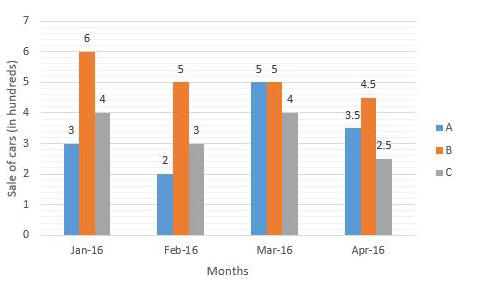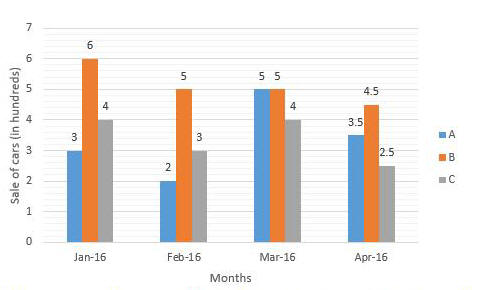# 09 July 2019 – Evening-Shift (Quantitative Aptitude)

SSC CHSL (Tier – 1) Online Exam Paper – 2018 “held on 09 JULY 2019”

Evening-Shift (Quantitative Aptitude)

Q1. If is an acute angle, and it is given that 5 sinΘ + 12 cosΘ = 13, then what is the value of tanΘ ?

Options:

1. 5/13
2. 12/13
3. 5/12
4. 13/12

Q2. If A, B and c can respectively complete a piece of work in 20,24 and 36 days respectively, how many days will they take to complete the work, if they work together ?

Options:

1. 360/43
2. 25/1
3. 37/4
4. 159/20

Q3. The given Bar Graph presents the scale (in 1000 kg) of a particular brand of tea by three outlets, A, B and C during the months Jan, Feb, Mar, and Apr, 2018.What is the ratio of rate of growth in sales from B to the rate of growth in sales from C in Mar 2018 with reference to its previous month ?

Options:

1. 10 : 21
2. 10 : 19
3. 9 : 19
4. 9: 16

Q4.  If I travel by bus, I reach my office 15 min late, and if I travel by car, I reach 10 min early. If the distance between my home and my office is 25 km, then the difference of the reciprocals of average speeds of the bus, in second per metre is:

Options:

1. 3/20
2. 3/50
3. 3/25
4. 3/10

Q5. The given Bar Graph presents the scale (in 1000 kg) of a particular brand of tea by three outlets, A, B and C during the months Jan, Feb, Mar, and Apr, 2018.What is the average scale per month by a during Jan-Mar, 2018 ?

Options:

1. 7505 kg
2. 7334.67 kg
3. 5500 kg
4. 7333.33 kg

Q6. The given Bar Graph presents the scale (in 1000 kg) of a particular brand of tea by three outlets, A, B and C during the months Jan, Feb, Mar, and Apr, 2018.Arrange the ratio of sales from B to that from A and C, taken together, month wise in ascending order

Options:

1. Jan, Mar, Feb, Apr
2. Jan, Feb, Mar, Apr
3. Jan, Mar, Apr, Feb
4. Jan, Apr, Mar, Feb

Correct answer: Jan, Mar, Apr, Feb

Q7. Which among the following increases continously in the range 0° < Θ < 90° ?

Options:

1. cosΘ
2. tanΘ
3. cotΘ
4. cosecΘ

Q8. If (3x + 1)³ + (x – 3)³ + (2x – 4)³ = 6(3x + 1) (x – 3) (x – 2), then x is equal to:

Options:

1. 1
2. 2
3. -1/3
4. 3

Q9. The value of the expression 1/4 {(a + 1/a)² – (a – 1/a)²} is :

Options:

1. 1
2. 4
3. 1/4
4. 1/2

Q10. Two items are sold for Rs.18,602, each. On one item there has been a gain of 31% and on the second item a loss of 29%. What was the overall loss or gain in the transaction?

Options:

1. Gain 8.25%
2. loss 7.91%
3. loss 8.25%
4. Gain 7.91%

Q11. An article is sold for Rs.688.16 after two successive discounts of 12% and 8% respectively. what is its marked price ?

Options:

1. Rs.900
2. Rs.850
3. Rs.820
4. Rs.800

Q12. The given Bar Graph presents the scale (in 1000 kg) of a particular brand of tea by three outlets, A, B and C during the months Jan, Feb, Mar, and Apr, 2018.by how much quantity is the average sale per month from B more or less than that from C ?

Options:

1. More by 1000 kg
2. Less by  1000 kg
3. More by 500 kg
4. Less by 800 kg

Correct answer: More by 1000 kg

Q13. In a stadium an athlete is running on a circular path with uniform speed during a practice session. The angle covered by him during one second is found to be 10° by a coach observing him from the centre of the circular track. What would be the measure of angle ( in degrees ) described by the athlete by an observer standing on the circle ?

Options:

1. It depends on the exact position of the observer on the circle
2. 5
3. 10
4. 20

Q14.  The simplified value of 7/3 of (3/5 ÷ 2/9 ) – (22/5 + 19/20  ÷ 1/2) is :

Options:

1. 1
2. 0
3. 1/2
4. 1/4

Q15. In a triangle ABC, PQ is a straight line parallel to AC, such that Area ABC : Area PBQ = 3 : 1 Then CB : CQ is equal to:

Options:

1. √3/2 (√3+1)
2. √3-2/2
3. √3/2 (√3-1)
4. √3/2

Q16. The circumcentre, incentre, orthocentre and the centroid of a triangle are one and the same point. The triangle must be:
(a) isosceles (b) right-angled (c) right-angled isosceles (d) equilateral

Options:

1. (c)
2. (a)
3. (d)
4. (b)

Q17. The average of a number and its reciprocal is 4. The average of its cube and its reciprocal is equal to:

Options:

1. 244
2. 288
3. 142
4. 256

Q18. For an examination of a practical based object, the total marks is 100. The break-up for theory, practical, project and viva voce is 40%, 30%, 20%, 10% . A candidate scored 80% in theory, 70% in practical, 60% in project and 50% in viva voce. What was her aggregate percentage ?

Options:

1. 72
2. 67
3. 68
4. 70

Q19. If  a : b : c = 1 : 3 : 5, what is the value of 4a – b + 2c / 3(a+b+c) ?

Options:

1. 10/27
2. 8/27
3. 1/3
4. 11/27

Q20. The simplified value of  1.0025 + 6.25 x 10¯6  / 0.0025 + 0.95 is :

Options:

1. 1.0505
2. 1.0525
3. 1.0005
4. 1.0025

Q21. The Seven digit number 78 x 1 y 68 is divisible by 88. The value of (x + y) is :

Options:

1. 13
2. 10
3. 14
4. 11

Q22.  For Θ being an acute angle it is given that, 3(cosec²Θ + cot² Θ) = 5. Then Θ is equal to :

Options:

1. 30°
2. 60°
3. 0°
4. 45°

Q23. The diagonals of a rhombus are respectively 4 cm and 12 cm. Its area (in cm²) is equal to :

Options:

1. 8
2. 36
3. 12
4. 24

Q24. Two chords AB and CD of a circle intersect at a point O inside the circle. It is given that AO = 1 cm, AB = 13 cm, CD = 8 cm. What is the ratio between the larger and smaller section among CO and OD

Options:

1. 5 : 3
2. 3 : 1
3. 11 : 5
4. 9 : 7